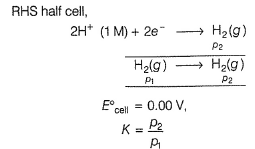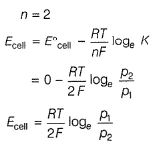Courses

# Retro (Past 13 Year) JEE Main (Electrochemistry)

## 29 Questions MCQ Test Chemistry for JEE Advanced | Retro (Past 13 Year) JEE Main (Electrochemistry)

Description
This mock test of Retro (Past 13 Year) JEE Main (Electrochemistry) for JEE helps you for every JEE entrance exam. This contains 29 Multiple Choice Questions for JEE Retro (Past 13 Year) JEE Main (Electrochemistry) (mcq) to study with solutions a complete question bank. The solved questions answers in this Retro (Past 13 Year) JEE Main (Electrochemistry) quiz give you a good mix of easy questions and tough questions. JEE students definitely take this Retro (Past 13 Year) JEE Main (Electrochemistry) exercise for a better result in the exam. You can find other Retro (Past 13 Year) JEE Main (Electrochemistry) extra questions, long questions & short questions for JEE on EduRev as well by searching above.
QUESTION: 1

### Only One Option Correct Type This section contains 29 multiple choice questions. Each question has four choices (a), (b), (c) and (d), out of which ONLY ONE is correct. Q. Two Faraday of electricity is passed through a solution of CuSO4. The mass of copper deposited at the cathode is (atomic mass of Cu = 63.5 amu) (JEE Main 2015)

Solution: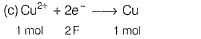Cu formed by two Faraday =
1 mole = 63.5 g

QUESTION: 2

### Resistance of 0.2 M solution of an electrolyte is 50 Ω. The specific conductance of the solution is 1.4 Sm-1. The resistance of 0.5 M solution of the same electrolyte is 280 Ω The molar conductivity of 0.5 Msolution of the electrolyte in S mol-1 is (JEE Main 2014)

Solution: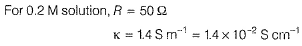The resistivity of the solution is related to specific conductance by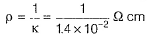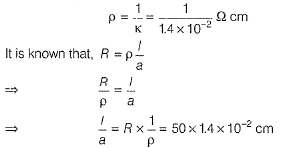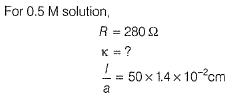The specific conductance of the solution is given by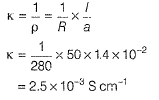Now, the molar conductivity of the solution is given by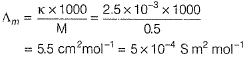Hence, the molar conductivity of 0.5 M solution of the electrolyte is 5 x 10 -4 S m2 mol-1.

QUESTION: 3

### The equivalent conductance of NaCI at concentration C and at infinite dilution are λc and λ∞ respectively. The correct relationship between λc and λ∞ is given as (JEE Main 2014)

Solution:

Debye-Huckel Onsager equation can be written as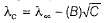Where,

λc = Molar conductivity of the solution at certain concentration
λ = Limiting molar conductivity
C = Concentration
B = Constant that depends on temperature, charges on the ions and dielectric constant as well as viscosity of the solution

QUESTION: 4

Given below are the half-cell reactions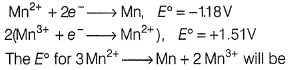(JEE Main 2014)

Solution: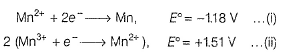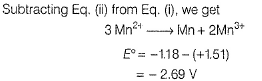Since, the value of E° is -ve, therefore the reaction is non-spontaneous.

QUESTION: 5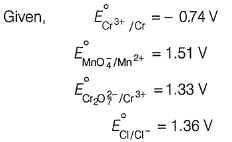Based on the data given above, strongest oxidising agent will be

(AIEEE 2013)

Solution:

Higher the SRP (Standard Reduction Potential), stronger is the oxidising agent. Among the given, electrode potentials,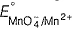is highest. Hence,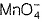is the strongest oxidising agent.

QUESTION: 6

The standard reduction potentials for Zn2+ / Zn, Ni2+ /Ni and Fe2+ / Fe are - 0.76, - 0.23 and - 0.44 V, respectively. The reaction X + y2+→ X2+ + Y will be spontaneous when

(AIEEE 2012)

Solution:

A cell reaction is spontaneous, if ΔG° < 0.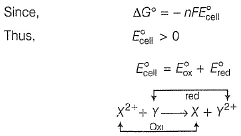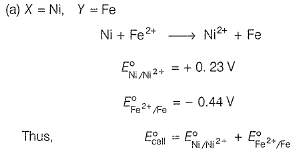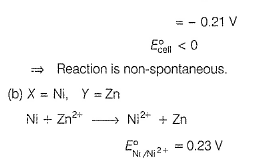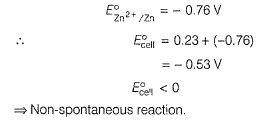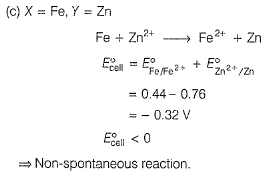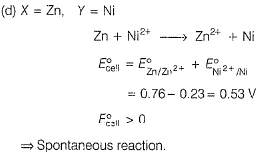QUESTION: 7

The reduction potential of hydrogen half-cell will be negative if

Solution:

For hydrogen electrode,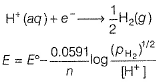Here, n = number of electrons involved in the reaction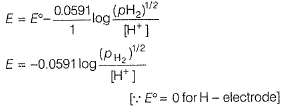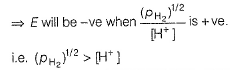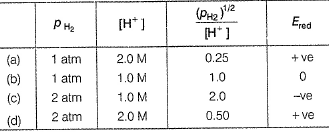Hence, when p(H2) is 2 atm and [H+] is 1.0 M, Ered is negative.

QUESTION: 8

Resistance of 0.2 M solution of an electrolyte is 50 Ω The specific conductance of the solution is 1.3 Sm-1. If resistance of the 0.4 M solution of the same electrolyte is 260 Ω, its molar conductivity is

(AIEEE 2011)

Solution: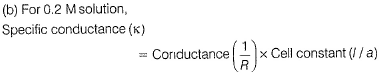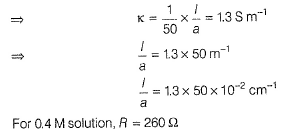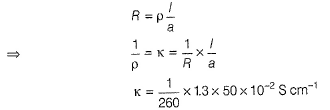Molar conductivity of the solution is given by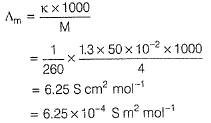QUESTION: 9

The Gibbs energy for the decomposition of Al2O3 at 500°C is as follows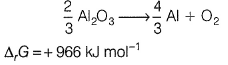The potential difference needed for electrolytic reduction of Al2O3 at 500°C is at least

(AIEEE 2010)

Solution: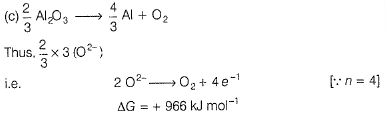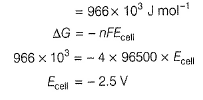QUESTION: 10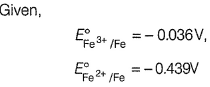The value of standard electrode potenital for the charge,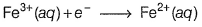(AIEEE 2009)

Solution:

It is given that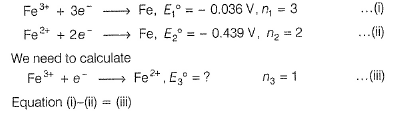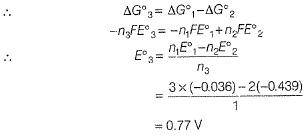QUESTION: 11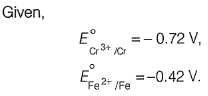The potential for the cell

Cr | Cr3+ (0.1 M) || Fe2+ (0.01 M) | Fe is

(AIEEE 2008)

Solution: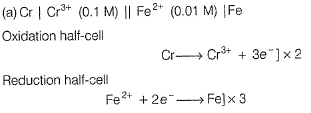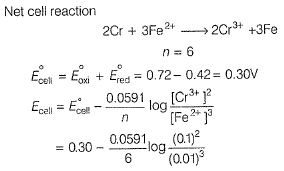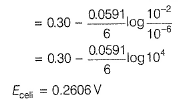QUESTION: 12

The equivalent conductances of two strong electrolytes at infinite dilution in H2O (where ions move freely through a solution) at 25°C a re given below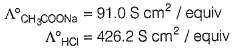What additional information /quantity one needs to calculate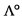of an aqueous solution of acetic acid?

Solution:

Molar conductivity of aqueous CH3COOH solution can be calculated from Kohlraush’s law as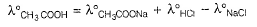Hence, for determining the molar conductivity of CH3COOH solution,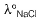is required.

QUESTION: 13

The cell, Zn | Zn2+ (1 M) || Cu2+ (1 M) | Cu

(E°cell = 1.10 V), was allowed to be completely discharged at 298 K. The relative concentration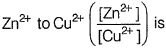(AIEEE 2007)

Solution:

Cell is completely discharged. It means equilibrium gets established, Ecell = 0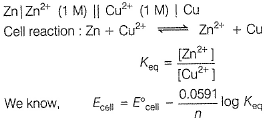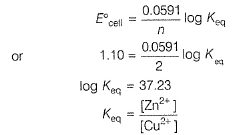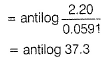QUESTION: 14

Resistance of a conductivity cell filled with a solution of an electrolyte of concentration 0.1 M is 100 Ω. The conductivity of this solution is 1.29 Sm-1. Resistance of the same cell when filled with 0.2 M of the same solution is 520 Ω. The molar conductivity of 0.2 M solution of the electrolyte will be

Solution:

For 0.1 M solution,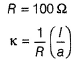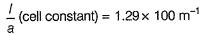For 0.2 M solution
Given, ft = 520 Ω, C = 0.2 M,
μ (molar conductivity) = ?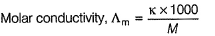k can be calculated as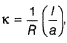now cell constant is known]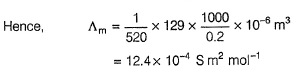QUESTION: 15

The molar conductivities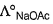and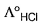at infinite dilution in water at 25°C are 91.0 and 426.2S cm2/mol, respectively. To calculate a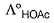additional value required is

(AIEEE 2006)

Solution:

According to Kohlrausch's law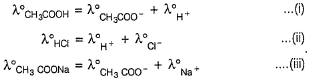Thus, on adding Eqs. (ii) and (ill),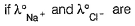subtracted, we can obtained the value of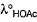. Thus, additional value required is λ°NaCl.

QUESTION: 16

Given the data at 25°C,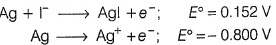What is the value of log Ksp for Agl?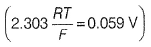(AIEEE 2006)

Solution: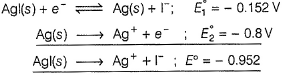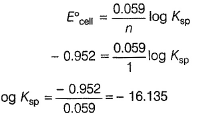QUESTION: 17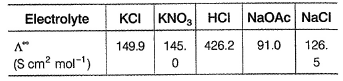Calculate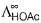using appropriate molar conductances of the electrolytes listed above at infinite dilution in H2O at 25°C

(AIEEE 2005)

Solution: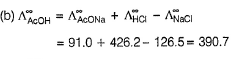QUESTION: 18

Aluminium oxide may be electrolysed at 1000°C to furnish aluminium metal (atomic mass = 27 u; 1 Faraday = 96500 C). The cathode reaction is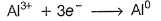To prepare 5.12 kg of aluminium metal by this method would require

(AIEEE 2005)

Solution: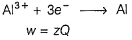where, w = amount of metal = 5.12 kg = 5.12 x 10g
z = electrochemical equivalent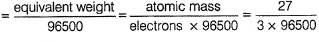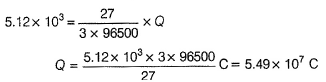QUESTION: 19

The limiting molar conductivities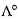for NaCI, KBr and KCI are 126, 152 and 150S cm2 mol-1, respectively . Thefor NaBr is

(AIEEE 2004)

Solution:

By Kohlrausch’s law.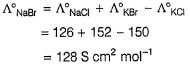QUESTION: 20

The E°m3+/m2+ values for Cr, Mn, Fe and Co are

- 0.41, + 1.57, + 0.77 and + 1.97 V,

respectively . For which one of these metals, the change in oxidation state from + 2 to + 3 is easiest?

(AIEEE 2004)

Solution: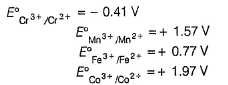More negative value of E°red indicates better reducing agent. Thus, easily oxidised. Hence, oxidation of Cr2+ to Cr3+ is the easiest.

QUESTION: 21

Consider the following Eo values :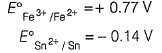Under standard conditions, the potential for the reaction,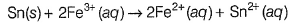(AIEEE 2004)

Solution: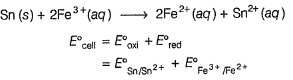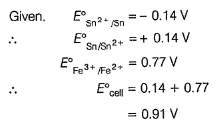QUESTION: 22

In a cell that utilises the reaction,
Zn (s)+ 2H + (aq) → Zn2+ (aq),+ H2(g)

addition of H2SO4 to cathode compartment will

(AIEEE 2004)

Solution: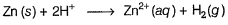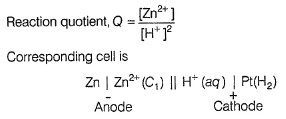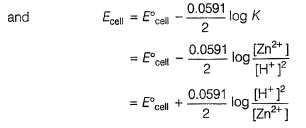If H2SO4 is added to cathodic compartment, (towards reactant side), then Q decrease (due to increase in H+).
Hence, equilibrium is displaced towards right and Ecell increases.

QUESTION: 23

The standard emf of a cell, involving one electron change is found to be 0.591 V at 25° C. The equilibrium constant of the reaction is (F= 96500 C mol-1)

(AIEEE2004)

Solution:

Relation between Keq and E°cell is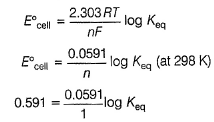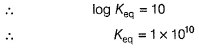QUESTION: 24

When during electrolysis of a solution of AgNO3, 9650 C of charge pass through the electroplating bath, the mass of silver deposited on the cathode will be

(AIEEE 2003)

Solution: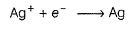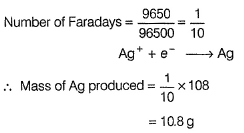QUESTION: 25

Standard reduction electrode potentials of three metals A, B and C are + 0.5 V, - 3.0 V and - 1.2 V, respectively. The reducing power of these metals are

(AIEEE 2003)

Solution:

More negative value of E° means higher reducing power.

QUESTION: 26

For a cell reaction involving a two electron change, the standard emf of the cell is found to be 0.295 V at 25°C. The equilibrium constant of the reaction at 25°C will be

(AIEEE 2003)

Solution:

The standard emf of the cell,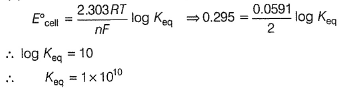QUESTION: 27

Conductivity (Seimen’s S) is directly proportional to area of the vessel and the concentration of the solution in it and is inversely proportional to the length of the vessel, then constant of proportionality is expressed in

(AIEEE 2002)

Solution: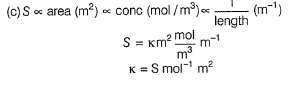QUESTION: 28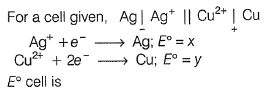(AIEEE 2002)

Solution:

At LHS (oxidation)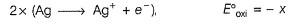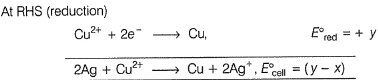Note E° values remain constant when half-cell equation is multiplied/divided.

QUESTION: 29

For the following cell with hydrogen electrodes at two different pressures p1 and p2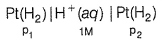emf is given by

(AIEEE 2002)

Solution:

LHS half-cell